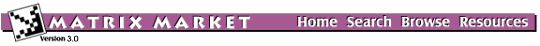S3DKT3M2: Finite element analysis of cylindrical shellsCylindrical shell, uniform 150x100 triangular mesh, R/t=1000

from set CYLSHELL, from the Independent Sets and Generators generated by MVMTLS

The following estimates were supplied by the author.

Max eigenvalue = 8.7984363691286471E+03 (revised 2 Jun 1998)
Min eigenvalue = 2.4269393992599454E-08
Condition number = 3.625322E+11 (revised 2 Jun 1998).

This matrix supercedes C3DKT3M2.

Help: My browser can't read the compressed data files. What now?

Visualizations

Click on image to get an enlarged version. Click on label to get an explanation.

Matrix Statistics

* * * * * * * * * * * * * * * * * * * * * * * * * * * * * * * * * * * * * *
* cyl shell 100 x 150 unif triang mesh R/t = 1000                         *
*                    Key = s3dkt3m2 , Type = RSA                          *
* * * * * * * * * * * * * * * * * * * * * * * * * * * * * * * * * * * * * *
*  Dimension N                                      =      90449  *
*  Number of nonzero elements                       =    1921955  *
*  Average number of nonzero elements/Column        =    21.2490  *
*  Standard deviation for above average             =     2.3992  *
*  Nonzero elements in strict lower part            =    1831506  *
*  Nonzero elements in strict upper part            =    1831506  *
*  Nonzero elements in main diagonal                =      90449  *
*  Weight of longest column                         =         47  *
*  Weight of shortest column                        =          9  *
*  Matching elements in symmetry                    =          0  *
*  Relative Symmetry Match (symmetry=1)             = NaN         *
*  Average distance of a(i,j)  from diag.           =  0.339E+03  *
*  Standard deviation for above average             =  0.299E+03  *
*-----------------------------------------------------------------*
*  Frobenius norm of A                              =  0.641E+06  *
*  Frobenius norm of symmetric part                 =  0.641E+06  *
*  Frobenius norm of nonsymmetric part              =  0.000E+00  *
*  Maximum element in A                             =  0.322E+04  *
*  Percentage of weakly diagonally dominant rows    =  0.000E+00  *
*  Percentage of weakly diagonally dominant columns =  0.000E+00  *
*-----------------------------------------------------------------*
*  Lower bandwidth  (max: i-j, a(i,j) .ne. 0)       =          0  *
*  Upper bandwidth  (max: j-i, a(i,j) .ne. 0)       =        614  *
*  Maximum Bandwidth                                =        615  *
*  Average Bandwidth                                =  0.609E+03  *
*  Number of nonzeros in skyline storage            =   55071893  *
*  90% of matrix is in the band of width            =       1219  *
*  80% of matrix is in the band of width            =       1215  *
*  The total number of nonvoid diagonals is         =        328  *
*  The 10 most important diagonals are (offsets)    :             *
*     0     1     2     3     4     5   603   604   605     6     *
*  The accumulated percentages they represent are   :             *
*   4.7   9.4  14.1  18.8  23.4  28.1  32.7  37.4  42.0  46.6     *
*-----------------------------------------------------------------*
*  The matrix does not have a block structure                     *
*-----------------------------------------------------------------*

Generator Information

Generator CYLSHELL
Source: Reijo Kouhia, Helsinki University of Technology, reijo.kouhia@hut.fi
Discipline:Structural mechanics
Accession:Summer 1997

The Matrix Market is a service of the Mathematical and Computational Sciences Division / Information Technology Laboratory / National Institute of Standards and Technology.

[ Home ] [ Search ] [ Browse ] [ Resources ]